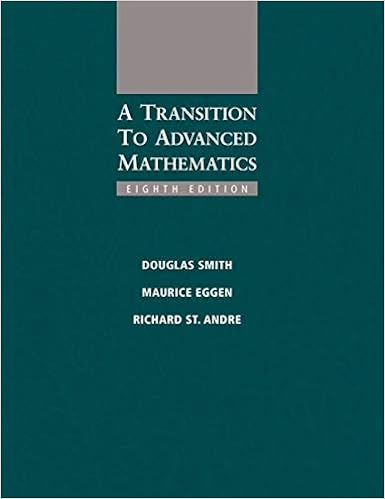# Solns_HW8_302_F18.pdf - Solutions to Written Problems on...

• 4

This preview shows page 1 - 3 out of 4 pages.

##### We have textbook solutions for you!
The document you are viewing contains questions related to this textbook.The document you are viewing contains questions related to this textbook.
Chapter 6 / Exercise 11
Smith/EggenExpert Verified
Solutions to Written Problems on Homework 8Problem 1.(a).Proof.In the following, we have used red to denote the justification of each equality from line to line.First we haveϕ((f, g) + (f0, g0))=ϕ((f+f0, g+g0))(Addition inR×S)=ϕ(f+f0, g+g0)( Notation change )=ϕXi0(ri+r0i)xi,Xi0(si+s0i)xi( Addition inR[x] andS[x] )=Xi0(ri+r0i, si+s0i)xi( Definition ofϕ)On the other hand, we haveϕ(f, g) +ϕ(f0, g0)=Xi0(ri, si)xi+Xi0(r0i, s0i)xi( Definition ofϕ)=Xi0((ri, si) + (r0i, s0i))xi( Addition in (R×S)[x] )=Xi0(ri+r0i, si+s0i)xi( Addition inR×S)Therefore we conclude thatϕ((f, g) + (f0, g0))=ϕ(f, g) +ϕ(f0, g0).(b).Proof.We haveiX=0(r, s)(r0i-, s0i-)=iX=0(rr0i-, ss0i-)( Multiplication inR×S)=(r0r0i, s0s0i) + (r1r0i-1, s1s0i-1) +· · ·+ (rr00, ss00)( Meaning ofiX=0)=(r0r0i+r1r0i-1+· · ·+rr00, s0s0i+s1s0i-1+· · ·+ss00)( Addition inR×S)=(iX=0rr0i-,iX=0ss0i-)( Meaning ofiX=0)=(ci, di).( Definition ofci, di)(c).Proof.Note that, by definition of multiplication inR[x] andS[x], we haveff0=i0cixiandgg0=i0gixi, withcianddias in part (b). We use this below.First we haveϕ((f, g)(f0, g0))=ϕ(ff0, gg0)(Multiplication inR×S)=ϕXi0cixi,Xi0dixi( Multiplication inR[x] andS[x] )=Xi0(ci, di)xi( Definition ofϕ)
##### We have textbook solutions for you!
The document you are viewing contains questions related to this textbook.The document you are viewing contains questions related to this textbook.
Chapter 6 / Exercise 11Expert Verified
•••